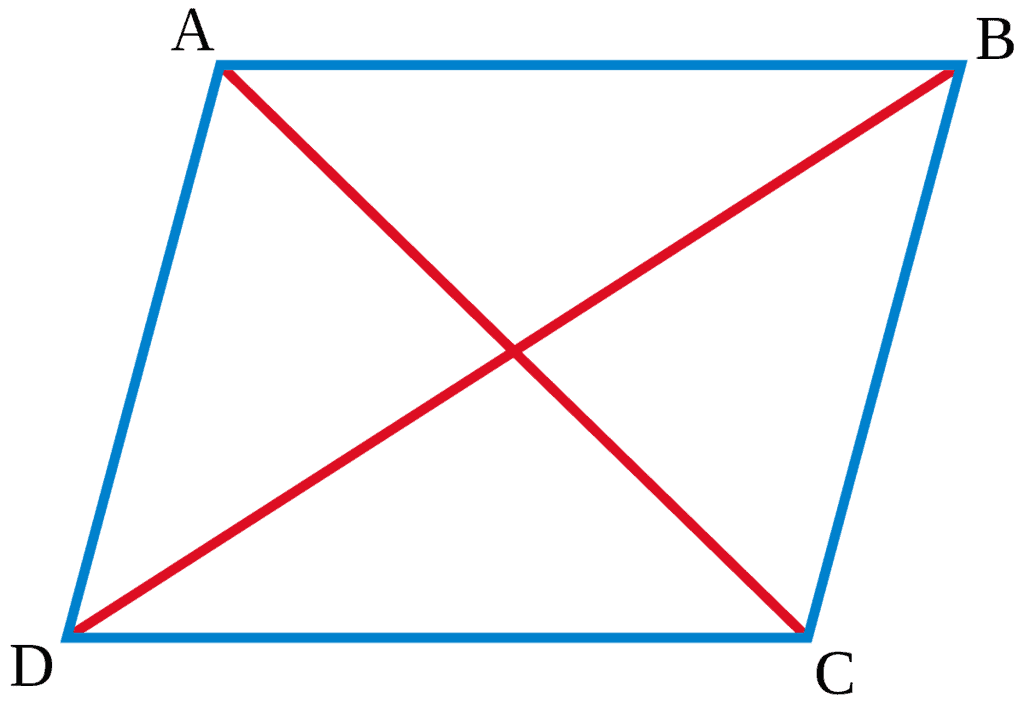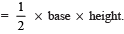# Facts that Matter- Areas of Parallelograms and Triangles Notes | Study Mathematics (Maths) Class 9 - Class 9

## Class 9: Facts that Matter- Areas of Parallelograms and Triangles Notes | Study Mathematics (Maths) Class 9 - Class 9

The document Facts that Matter- Areas of Parallelograms and Triangles Notes | Study Mathematics (Maths) Class 9 - Class 9 is a part of the Class 9 Course Mathematics (Maths) Class 9.
All you need of Class 9 at this link: Class 9

Areas of Plane Figures• Two congruent figures have equal areas.
• A diagonal of a parallelogram divides it into two triangles of equal area.
• Parallelograms on the same base (or equal base) and between the same parallels are equal in area.
• Triangles on the same base (or equal bases) and between the same parallels are equal in area.
• Area of a parallelogram = base x height.
• Area of a triangle• A median of a triangle divides it into two triangles of equal area.
• Diagonals of a parallelogram divides it into four triangles of equal area.

Remember:

• The part of the plane enclosed by a simple closed figure is called a planar region.
• The measure of a planar region is called its area.
• Two figures are called congruent, if they have the same shape and the same size.
• If two plane figures are congruent, they have equal areas.
• Two plane figures having equal areas need not to be congruent.

Figures on the same Base and between the same Parallels
Two figures are said to be on the same base and between the same parallels, if they have a common base and vertices (or vertex) opposite to the common base of each figure lie on a line parallel to the base.

Note:
Out of the two parallels one must be the line containing the common base.

The document Facts that Matter- Areas of Parallelograms and Triangles Notes | Study Mathematics (Maths) Class 9 - Class 9 is a part of the Class 9 Course Mathematics (Maths) Class 9.
All you need of Class 9 at this link: Class 9Use Code STAYHOME200 and get INR 200 additional OFF

## Mathematics (Maths) Class 9

73 videos|352 docs|109 tests

Track your progress, build streaks, highlight & save important lessons and more!

,

,

,

,

,

,

,

,

,

,

,

,

,

,

,

,

,

,

,

,

,

;